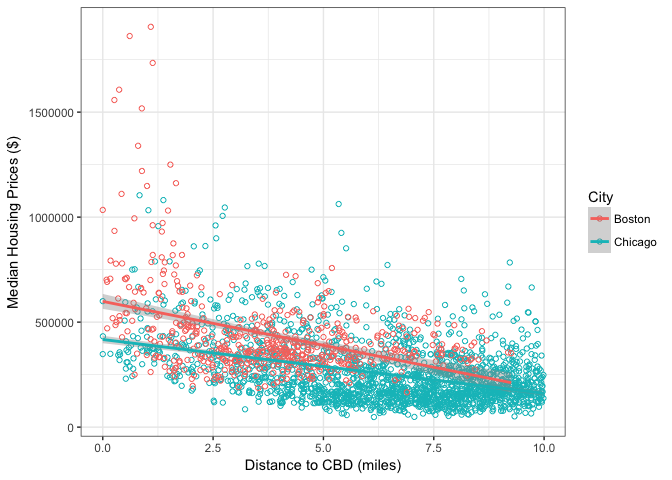## A Simple Spatial Equilibrium Model with Tidycensus

The Spatial Equilibrium concept is well known to urban economists. In a nutshell, it states that in equilibrium there are no rents to be gained by changing locations. Ed Glaeser begins Chapter 2 of his book: “Cities, Agglomeration, and Spatial Equilibrium” with the well known Alonso-Muth-Mills model. In this post, I want to summarize it briefly following Ed Glaeser presentation and reproduce his 2.1. and 2.2. figures. This is the perfect excuse to play around with the “tidycensus” package.

## Spatial equilibrium in the Alonso-Muth-Mills model

In its simplest form, the model assumes a monocentric closed city. Furthermore, we assume that there are $N$ city dwellers that maximize their utility which depends on $C$ consumption and $L$ units of land

$\underset{C}{\mathrm{max}\,}U(C,L)$

City dwellers use $L$ units of land, consume $C$, and commute to the city center. Consumption is equal to their income, minus transport costs and rental costs per unit of land. City dwellers income is an exogenous wage w. Commuting costs are a function of distance and for simplicity we assume it linear $t(d)=td$. Rental costs per unit of land are $r(d)L$, where $r(d)$ is the rental cost per unit of land. Thus,

$C = w − td − r(d)L$

To get to the spatial equilibrium we first rewrite utility function

$\underset{d}{\mathrm{max}}\,U( w -td -r(d) L,L)$

Then, the first order conditions imply

$\frac{\partial U}{\partial d}(-t-\frac{\partial r}{\partial d} L )=0$ $r′= − \frac{t}{L}$

The equilibrium condition states that rents must decline exactly to offset the increase in transportation cost. The simple assumption that commuting costs are linear implies that the rent gradient is linear:

$r(d)=r(0) -\frac{td}{L}$

The key prediction of the model is that land rents will decline with distance to the city center.

## R code

Let’s take the model to the data and reproduce figures 2.1. and 2.2 of “Cities, Agglomeration, and Spatial Equilibrium”. The focus are two cities, Chicago and Boston. These cities are chosen because both differ in how easy is to access to their city centers. Chicago is fairly easy, Boston is more complicated. Our model then implies that gradients then should reflect the differential costs to access the city centers.

So let’s begin, the first step is to get some data. To do so I’m are going to use the “tidycensus” package. This package will allow me to get data from the census website using their API. We are also going to need the help of three other packages: “sf” to handle spatial data, “dplyr” my go-to package to wrangle data, and “ggplot2” to plot my results.

require("tidycensus", quietly=TRUE)
require("sf", quietly=TRUE)
require("dplyr", quietly=TRUE)
require("ggplot2", quietly=TRUE)


In order to get access to the Census API, I need to supply a key, which can be obtained from http://api.census.gov/data/key_signup.html.

census_api_key("YOUR CENSUS API HERE")

## To install your API key for use in future sessions, run this function with install = TRUE.


Let’s get Median Housing Values from the latest ACS. I’ll use the variable “B25077_001E” which is the estimated Median Value of Owner-Occupied Housing Units. The package “tidycensus” provides the get_acs function that will let me retrieve this variable for the county at the block group level. It is important to note the option geometry=T. This option downloads also geometry features of the census block that will allow later on to compute distances.

chicago<-get_acs(geography = "block group", variables = "B25077_001E",  state = "IL",county = "Cook County",year=2016,geometry = T) #retrieve median housing values for Cook County

boston<-get_acs(geography = "block group", variables = "B25077_001E",  state = "MA",county = "Suffolk County",year=2016,geometry = T)   #retrieve median housing values for Suffolk County


Next, we need the city centers. I create two “sf” objects that have the coordinates to the city center.

chicago_cbd<-st_as_sf(x = read.table(text="-87.627800  41.881998"),
coords = c(1,2),
crs = "+proj=longlat +datum=WGS84")

coords = c(1,2),
crs = "+proj=longlat +datum=WGS84")


Now, I need to put everything in the same projection. I re-project my cbds to the same projection as my tibbles.

chicago_cbd <-chicago_cbd %>% st_transform(st_crs(chicago) )
boston_cbd <-boston_cbd %>% st_transform(st_crs(boston) )


The next step is to create distances. For that, I use the st_distance function. Given the projection of my tibbles is in meters, I transform all my distances to miles, one meter is 0.000621371 miles.

chicago$dist_CBD<-st_distance(chicago,chicago_cbd) #computes distance to CBD (in meters) chicago$dist_CBD<-as.numeric(chicago$dist_CBD)*0.000621371 #change units to miles boston$dist_CBD<-st_distance(boston,boston_cbd) #computes distance to CBD (in meters)
boston$dist_CBD<-as.numeric(boston$dist_CBD)*0.000621371 #change units to miles


The last part is some cleaning up. I add a column with the name of the city, keep block groups in Cook County that are within 10 miles of the city center, and keep the data.frames from my tibbles,. Finally, I bind the data.frames together.

boston$City<-"Boston" chicago$City<-"Chicago"

chicago<-chicago %>% filter(dist_CBD<=10)
chicago<-data.frame(chicago)
boston<-data.frame(boston)

dta<-rbind(chicago,boston)


We are now ready to plot. I use a regular scatter plot with hollow circles and add a linear regression line on a black and white background (theme_bw())

ggplot(dta, aes(x=dist_CBD, y=estimate, color=City)) +
geom_point(shape=1) +    # Use hollow circles
geom_smooth(method=lm) +  # Add linear regression line
xlab("Distance to CBD (miles)") +
ylab("Median Housing Prices (\$)") +
theme_bw()Conclusion: the data reflect the predictions of the simple Alonso-Muth-Mills model, land rents will decline with distance to the city center. The speed at which they decline depends on transportation costs.

## Session Info

sessionInfo()

R version 3.5.0 (2018-04-23)
Platform: x86_64-apple-darwin15.6.0 (64-bit)
Running under: macOS High Sierra 10.13.5

Matrix products: default
BLAS: /Library/Frameworks/R.framework/Versions/3.5/Resources/lib/libRblas.0.dylib
LAPACK: /Library/Frameworks/R.framework/Versions/3.5/Resources/lib/libRlapack.dylib

locale:
 en_US.UTF-8/en_US.UTF-8/en_US.UTF-8/C/en_US.UTF-8/en_US.UTF-8

attached base packages:
 stats     graphics  grDevices utils     datasets  methods   base

other attached packages:
 bindrcpp_0.2.2   ggplot2_2.2.1    dplyr_0.7.4      sf_0.6-3
 tidycensus_0.4.6

loaded via a namespace (and not attached):
 Rcpp_0.12.16     plyr_1.8.4       pillar_1.2.2     compiler_3.5.0
 bindr_0.1.1      class_7.3-14     tools_3.5.0      uuid_0.1-2
 digest_0.6.15    gtable_0.2.0     jsonlite_1.5     evaluate_0.10.1
 tibble_1.4.2     lattice_0.20-35  pkgconfig_2.0.1  rlang_0.2.0
 DBI_0.8          curl_3.2         rgdal_1.2-18     yaml_2.1.18
 spData_0.2.8.3   e1071_1.6-8      httr_1.3.1       stringr_1.3.0
 knitr_1.20       xml2_1.2.0       hms_0.4.2        rappdirs_0.3.1
 tigris_0.7       tidyselect_0.2.4 classInt_0.2-3   rprojroot_1.3-2
 grid_3.5.0       glue_1.2.0       R6_2.2.2         foreign_0.8-70
 purrr_0.2.5      udunits2_0.13    magrittr_1.5     scales_0.5.0
 backports_1.1.2  htmltools_0.3.6  units_0.5-1      maptools_0.9-2
 assertthat_0.2.0 rvest_0.3.2      colorspace_1.3-2 labeling_0.3
 stringi_1.1.7    lazyeval_0.2.1   munsell_0.4.3    lwgeom_0.1-4